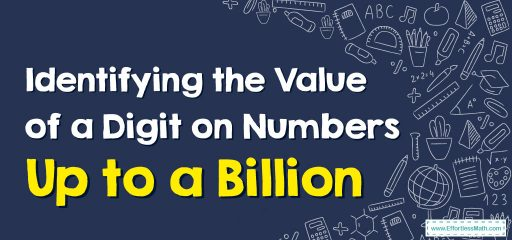# How to Identify the Value of a Digit on Numbers Up to a Billion

To identify the value of a digit in numbers up to a billion, it's essential to understand the place value system. The place value system is based on powers of 10, where each position represents a different power of 10.This place value chart shows you all of the places that lead up to billions.

Whenever one thinks of value, they are discussing how much the digit is worth in that number. For instance, the value of a ‘6 ‘ within the tens place is 60.

Here’s a step-by-step guide to help you identify the value of a digit in numbers up to a billion:

1. Write down the number.

For example, let’s consider the number 345,678,912.

1. Identify the position of the digit in question.

Counting from right to left, assign a place value to each digit in the number:

• Ones: 1st position
• Tens: 2nd position
• Hundreds: 3rd position
• Thousands: 4th position
• Ten thousands: 5th position
• Hundred thousands: 6th position
• Millions: 7th position
• Ten millions: 8th position
• Hundred millions: 9th position
1. Determine the place value based on the digit’s position.

Let’s say we want to identify the value of the digit ‘6’ in our example number. Since it’s in the 5th position from the right, it has a place value of ten thousands.

1. Calculate the value of the digit.

To calculate the value of the digit ‘6’ in the ten thousands position, multiply the digit by its place value (10,000):

6 x 10,000 = 60,000

So, the value of the digit ‘6’ in the number 345,678,912 is 60,000.

Repeat the process for any digit in the number, up to a billion.

## A step-by-step guide to identifying value of a digit on numbers up to a billion

### Example 1:

What is the place of 5 and 1 in number 5,601,754 ?

Solution: Use place-value chart to find out the place of each number.

Therefore, 5 is in the millions place and 1 is in the thousands place.

### What people say about "How to Identify the Value of a Digit on Numbers Up to a Billion - Effortless Math: We Help Students Learn to LOVE Mathematics"?

No one replied yet.

X
30% OFF

Limited time only!

Save Over 30%

SAVE $5 It was$16.99 now it is \$11.99